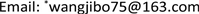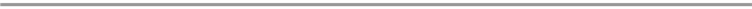﻿ 基于截断学习效应的共同工期指派调度问题研究 Research on Common Due Date Assignment Scheduling Problem with Truncated Learning Effect

Vol. 09  No. 03 ( 2020 ), Article ID: 34542 , 5 pages
10.12677/AAM.2020.93040

Research on Common Due Date Assignment Scheduling Problem with Truncated Learning Effect

Changlin Zhao, Tao Wu, Yuhao Ma, Liqiang Zhang, Jianqiang Wu, Jibo Wang*

School of Science, Shenyang Aerospace University, Shenyang LiaoningReceived: Feb. 25th, 2020; accepted: Mar. 9th, 2020; published: Mar. 16th, 2020ABSTRACT

This paper studies a single machine scheduling problem with truncated learning effect, where the actual processing time of a job depends on the position and truncation factor. Under the slack due date assignment, the objective is to determine the schedule of jobs and the common due date in order to minimize the linear weighted sum of the earliness, tardiness, common due date and total completion time, where the weights of the earliness and tardiness are positional weights. We prove that the problem can be solved in polynomial time.

Keywords:Scheduling, Learning Effect, Common Due Date Assignment1. 引言

2. 问题描述

${p}_{ih}={\stackrel{¯}{p}}_{i}\mathrm{max}\left\{{h}^{{a}_{i}},{b}_{i}\right\},\text{\hspace{0.17em}}i,h=1,2,\cdots ,n,$ (1)

$F\left(\pi ,d\right)=\underset{i=1}{\overset{n}{\sum }}{w}_{i}|{C}_{\pi \left(i\right)}-d|+{w}_{0}d+{w}_{n+1}\underset{i=1}{\overset{n}{\sum }}{C}_{\pi \left(i\right)}$ (2)

$1|CON,TLE|\underset{i=1}{\overset{n}{\sum }}{w}_{i}|{C}_{\pi \left(i\right)}-d|+{w}_{0}d+{w}_{n+1}\underset{i=1}{\overset{n}{\sum }}{C}_{\pi \left(i\right)},$ (3)

3. 主要结论

${\vartheta }_{i}=\left\{\begin{array}{l}{w}_{0}+\underset{l=1}{\overset{i-1}{\sum }}{w}_{l}+\left(n-i+1\right){w}_{n+1},i=1,2,\cdots ,k\\ \underset{l=i}{\overset{n}{\sum }}{w}_{l}+\left(n-i+1\right){w}_{n+1},i=k+1,k+2,\cdots ,n\end{array}$ (4)

$\begin{array}{l}F\left(\pi ,d\right)=\underset{i=1}{\overset{n}{\sum }}{w}_{i}|{C}_{\pi \left(i\right)}-d|+{w}_{0}d+{w}_{n+1}\underset{i=1}{\overset{n}{\sum }}{C}_{\pi \left(i\right)}\\ \text{}=\underset{i=1}{\overset{k-1}{\sum }}{w}_{i}\left({C}_{\pi \left(k\right)}-{C}_{\pi \left(i\right)}\right)+\underset{i=k+1}{\overset{n}{\sum }}{w}_{i}\left({C}_{\pi \left(i\right)}-{C}_{\pi \left(k\right)}\right)+{w}_{0}{C}_{\pi \left(k\right)}+{w}_{n+1}\underset{i=1}{\overset{n}{\sum }}{C}_{\pi \left(i\right)}\\ \text{}=\underset{i=1}{\overset{k-1}{\sum }}{w}_{i}\left(\underset{l=i+1}{\overset{k}{\sum }}{p}_{\pi \left(l\right)}^{A}\right)+\underset{i=k+1}{\overset{n}{\sum }}{w}_{i}\left(\underset{l=k+1}{\overset{i}{\sum }}{p}_{\pi \left(l\right)}^{A}\right)+{w}_{0}\underset{l=1}{\overset{k}{\sum }}{p}_{\pi \left(l\right)}^{A}+{w}_{n+1}\underset{i=1}{\overset{n}{\sum }}\left(n-i+1\right){p}_{\pi \left(i\right)}^{A}\\ \text{}=\underset{i=1}{\overset{n}{\sum }}{\vartheta }_{i}{p}_{\pi \left(i\right)}^{A}\end{array}$

$F\left(\pi ,d\right)=\underset{i=1}{\overset{n}{\sum }}{\vartheta }_{i}{p}_{\pi \left(i\right)}^{A}=\underset{i=1}{\overset{n}{\sum }}{\vartheta }_{i}{\stackrel{¯}{p}}_{\pi \left(i\right)}\mathrm{max}\left\{{i}^{{a}_{\pi \left(i\right)}},{b}_{\pi \left(i\right)}\right\}$ (5)

${C}_{ih}={\vartheta }_{h}{\stackrel{¯}{p}}_{i}\mathrm{max}\left\{{h}^{{a}_{i}},{b}_{i}\right\}$ (6)

${x}_{ih}=\left\{\begin{array}{l}1,如果工件{J}_{i}排在第h个位置,\\ 0,否则,\end{array}$ (7)

$\mathrm{min}\underset{i=1}{\overset{n}{\sum }}\underset{h=1}{\overset{n}{\sum }}{C}_{ih}{x}_{ih}$ (8)

s.t.

$\underset{h=1}{\overset{n}{\sum }}{x}_{ih}=1,i=1,2,\cdots ,n$ (9)

$\underset{i=1}{\overset{n}{\sum }}{x}_{ih}=1,h=1,2,\cdots ,n$ (10)

${x}_{ih}=0或1,\text{\hspace{0.17em}}i,h=1,2,\cdots ,n$ (11)

$F\left(\pi ,d\right)=\underset{i=1}{\overset{n}{\sum }}{\vartheta }_{i}{p}_{\pi \left(i\right)}^{A}=\underset{i=1}{\overset{n}{\sum }}{\vartheta }_{i}{\stackrel{¯}{p}}_{\pi \left(i\right)}\mathrm{max}\left\{{i}^{a},b\right\}$ (12)

${\beta }_{i}={\vartheta }_{i}\mathrm{max}\left\{{i}^{a},b\right\}$，则 $F\left(\pi ,d\right)=\underset{i=1}{\overset{n}{\sum }}{\vartheta }_{i}{p}_{\pi \left(i\right)}^{A}=\underset{i=1}{\overset{n}{\sum }}{\beta }_{i}{\stackrel{¯}{p}}_{\pi \left(i\right)}$，由Hardy等  得，式子 $\underset{i=1}{\overset{n}{\sum }}{\beta }_{i}{\stackrel{¯}{p}}_{\pi \left(i\right)}$ 的最小值，能够通过两个序列 $\left\{{\beta }_{i}\right\}$$\left\{{\stackrel{¯}{p}}_{\pi \left(i\right)}\right\}$ 的相反序列匹配得到。

Research on Common Due Date Assignment Scheduling Problem with Truncated Learning Effect[J]. 应用数学进展, 2020, 09(03): 341-345. https://doi.org/10.12677/AAM.2020.93040

1. 1. Biskup, D. (2008) A State-of-the-Art Review on Scheduling with Learning Effects. European Journal of Operational Research, 188, 315-329. https://doi.org/10.1016/j.ejor.2007.05.040

2. 2. Azzouz, A., Ennigrou, M. and Said, L.B. (2018) Scheduling Problems under Learning Effects: Classification and Cartography. International Journal of Production Research, 56, 1642-1661. https://doi.org/10.1080/00207543.2017.1355576

3. 3. Cheng, T.C.E., Wu, C.-C., Chen, J.-C., Wu, W.-H. and Cheng, S.-R. (2013) Two-Machine Flowshop Scheduling with a Truncated Learning Function to Minimize the Makespan. International Journal of Production Economics, 141, 79-86. https://doi.org/10.1016/j.ijpe.2012.03.027

4. 4. 王雪茹, 白雪莲, 王吉波, 殷娜. 基于截断学习效应的加权总完工时间流水作业排序问题研究[J]. 重庆师范大学学报, 2017, 34(5): 12-17.

5. 5. Wang, X.-R., Jin, J., Wang, J.-B. and Ji, P. (2014) Single Machine Scheduling with Truncated Job-Dependent Learning Effect. Optimization Letters, 8, 669-677. https://doi.org/10.1007/s11590-012-0579-0

6. 6. Wang, J.-B., Liu, F. and Wang, J.-J. (2019) Research on m-Machine Flow Shop Scheduling with Truncated Learning Effects. International Transactions in Operational Research, 26, 1135-1151. https://doi.org/10.1111/itor.12323

7. 7. Graham, R.L., Lawler, E.L., Lenstra, J.K. and Rinnooy Kan, A.H.G. (1979) Optimization and Approximation in Deterministic Sequencing and Scheduling: A Survey. Annals of Discrete Mathematics, 5, 287-326. https://doi.org/10.1016/S0167-5060(08)70356-X

8. 8. Brucker, P. (2001) Scheduling Algorithms. 3rd Edition, Springer, Berlin. https://doi.org/10.1007/978-3-662-04550-3

9. 9. Hardy, G.H., Littlewood, J.E. and Polya, G. (1934) Inequalities. Cambridge University Press, Cambridge.